Do you like the article?
Share it with others —
Use new possibilities of MetaTrader 5

#### Similar articles# Creating a Multi-Currency Multi-System Expert Advisor

MetaTrader 5Examples | 5 December 2013, 11:0732 14111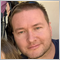### Introduction

I believe there are quite a few traders who trade more than one trading symbol and use multiple strategies. This approach does not only allow you to potentially increase your profit but to also minimize the risk of substantial drawdown upon efficient money management. When creating an Expert Advisor, the first natural step in checking the efficiency of the program strategy is optimization in order to determine the best input parameters.

With parameter values identified, Expert Advisors would technically be ready for trading. However that would leave one important question unanswered. What would testing results be like if a trader could put all his strategies together in a single Expert Advisor? The realization that drawdown on several symbols or strategies might at some point overlap and result in a ghastly total drawdown or even a margin call may sometimes come as a nasty surprise.

This article introduces a concept of creating a multi-currency multi-system Expert Advisor that will allow us to find an answer to this important question.

### 1. Structure of the Expert Advisor

In general terms, the structure of the Expert Advisor is as follows: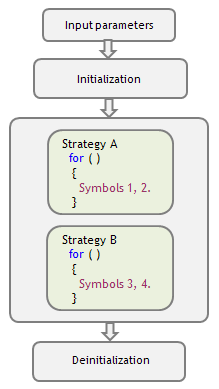Fig. 1. Structure of the multi-currency multi-system Expert Advisor

As you can see, the program is based on a for loop. Each strategy is arranged in a loop where each iteration is responsible for trading each symbol separately. Here, you can arrange in loops unlimited number of strategies. Important is for your computer to have sufficient resources to "process" such a program.

You should keep in mind that there may only be one position for each traded symbol in MetaTrader 5. Such position represents the sum of lots of previously executed Buys and Sells. Therefore, the result of multi-strategy testing for one symbol will not be identical to the sum of separate testing results of the same strategies for the same symbol.

For a closer consideration of the structure of the Expert Advisor we will take 2 strategies each of which trades two symbols:

Strategy A:

• Buy: Ask price reaches the lower band of the Bollinger Bands indicator calculated based on Low price.
Closing: Bid price reaches the lower band of the Bollinger Bands indicator calculated based on High price.
• Sell: Bid price reaches the upper band of the Bollinger Bands indicator calculated based on High price.
Closing: Ask price reaches the upper band of the Bollinger Bands indicator calculated based on Low price.
• Restriction: only one deal can be executed on any given bar.

Strategy В:

• Buy: the previous bar is bearish (close < open) and Ask price reaches the previous bar's high.
Closing: by Stop Loss or Take Profit.
• Sell: the previous bar is bullish (close > open) and Bid price reaches the previous bar's low.
Closing: by Stop Loss or Take Profit.
• Restriction: only one deal can be executed on any given bar.

To be independent from the new ticks for a symbol on which the Expert Advisor will be tested or which it will trade, it is advisable to use the OnTimer() function for trading in multi-currency mode.

For this purpose, when initializing the Expert Advisor we specify the frequency of generating an event for program calculation call using the EventSetTimer() function, and upon deinitialization we use the EventKillTimer() function to tell the terminal to stop generation of events:

```// Include standard libraries
// Create external parameters
// Create arrays, variables, indicator handles, etc.

//--- Initialization of the Expert Advisor
int OnInit()
{
//--- Set event generation frequency
EventSetTimer(1); // 1 second
// ...
return(0);
}
void OnTimer()
{
// ...
}
//--- Deinitialization of the Expert Advisor
void OnDeinit(const int reason)
{
//--- Stop event generation
EventKillTimer();
// ...
}
```

Instead of EventSetTimer(), you can also use EventSetMillisecondTimer(), where frequency is set accurate to millisecond but you should not misuse it by too frequent program calculation calls.

For access to account, position and symbol settings, as well as trading functions, we will use CAccountInfo, CPositionInfo, CSymbolInfo and CTrade classes, respectively. Let's include them in the Expert Advisor:

```//--- Include standard libraries
```

Since the Expert Advisor is based on for loops, we will need to create arrays for its external parameters. Let's first create constants equal to the number of symbols for each strategy:

```//--- Number of traded symbols for each strategy
#define Strategy_A 2
#define Strategy_B 2
```

We then create external parameters. Using constants, we determine sizes of arrays to which they will be copied. Further, we create indicator handles and other global variables.

An example for one symbol of strategy А is provided below:

```//------------------- External parameters of strategy A
input string          Data_for_Strategy_A="Strategy A -----------------------";
//--- Symbol 0
input string          Symbol_A0      = "EURUSD";   // Symbol
//--- Bollinger Bands (BB) parameters
input ENUM_TIMEFRAMES Period_A0      = PERIOD_H1;  // ВВ period
input uint            BBPeriod_A0    = 20;         // Period for calculation of the moving average of BB
input int             BBShift_A0     = 0;          // Horizontal shift of ВВ
input double          BBDeviation_A0 = 2.0;        // Number of standard deviations of BB
//...
//--- General parameters of strategy A
input double          DealOfFreeMargin_A = 1.0;    // Percent of free margin for a deal
input uint            MagicNumber_A      = 555;    // Magic number
input uint            Slippage_A         = 100;    // Permissible slippage for a deal
//...
//------------- Set variables of strategy A -----
//--- Arrays for external parameters
string          Symbol_A[Strategy_A];
ENUM_TIMEFRAMES Period_A[Strategy_A];
int             BBPeriod_A[Strategy_A];
int             BBShift_A[Strategy_A];
double          BBDeviation_A[Strategy_A];
//--- Arrays for global variables
double          MinLot_A[Strategy_A],MaxLot_A[Strategy_A];
double          Point_A[Strategy_A],ContractSize_A[Strategy_A];
uint            DealNumber_A[Strategy_A];
datetime        Locked_bar_time_A[Strategy_A],time_arr_A[];
//--- Indicator handles
int             BB_handle_high_A[Strategy_A];
int             BB_handle_low_A[Strategy_A];
//--- Arrays for indicator values
double          BB_upper_band_high[],BB_lower_band_high[];
double          BB_upper_band_low[],BB_lower_band_low[];
//--- Class
//...
//--- Set global variables for all strategies
long            Leverage;
//--- Classes
CAccountInfo    AccountInfo;
CPositionInfo   PositionInfo;
CSymbolInfo     SymbolInfo;
```

To have the possibility to disable trading for a certain symbol, we have created a Boolean variable IsTrade_A0 that will be placed at the very beginning of for loops.

### 2. Initialization of the Expert Advisor

First, let's get the values required for all strategies, e.g. leverage. Since leverage is applied to the trading account and has nothing to do with a strategy or a symbol, there is no need to copy its value to the arrays:

```//--- Get the leverage for the account
Leverage=AccountInfo.Leverage();
```

We then copy external variables to arrays.

```//--- Copy external variables to arrays
Symbol_A     =Symbol_A0;
Period_A     =Period_A0;
BBPeriod_A   =(int)BBPeriod_A0;
BBShift_A    =BBShift_A0;
BBDeviation_A=BBDeviation_A0;
```

If any external parameter is defined by the type that will require conversion to another one, this can be done in a more convenient way when copying to arrays.

In this case, we can see that BBPeriod_A0 was created as uint to prevent the user from setting a negative value. Here, we convert it to int and copy it to the array which was also created as int. Otherwise, the compiler will give a warning if you try to insert uint type parameter in the indicator handle.

Let's further see whether the traded symbol is available in the Market Watch and whether it has been used more than once within one strategy:

```//--- Check for the symbol in the Market Watch
for(int i=0; i<Strategy_A; i++)
{
if(IsSymbolInMarketWatch(Symbol_A[i])==false)
{
Print(Symbol_A[i]," could not be found on the server!");
ExpertRemove();
}
}

//--- Check whether the symbol is used more than once
if(Strategy_A>1)
{
for(int i=0; i<Strategy_A-1; i++)
{
for(int j=i+1; j<Strategy_A; j++)
{
if(Symbol_A[i]==Symbol_A[j])
{
Print(Symbol_A[i]," is used more than once!");
ExpertRemove();
}
}
}
}
//--- The IsSymbolInMarketWatch() function
bool IsSymbolInMarketWatch(string f_Symbol)
{
for(int s=0; s<SymbolsTotal(false); s++)
{
if(f_Symbol==SymbolName(s,false))
return(true);
}
return(false);
}
```

If the symbols were selected correctly, check for errors in input parameters for each of them, create indicator handles, get the data required for the lot calculation and, if necessary, do other things as defined by the given strategy.

We will implement the above mentioned actions inside a for loop.

```//--- General actions
for(int i=0; i<Strategy_A; i++)
{
//--- Check for errors in input parameters
//...
//--- Set indicator handles
BB_handle_high_A[i]=iBands(Symbol_A[i],Period_A[i],BBPeriod_A[i],BBShift_A[i],BBDeviation_A[i],
PRICE_HIGH);
if(BB_handle_high_A[i]<0)
{
Print("Failed to create a handle for Bollinger Bands based on High prices for ",Symbol_A[i]," . Handle=",INVALID_HANDLE,
"\n Error=",GetLastError());
ExpertRemove();
}
//...
//--- Calculate data for the Lot
//--- set the name of the symbol for which the information will be obtained
SymbolInfo.Name(Symbol_A[i]);
//--- minimum and maximum volume size in trading operations
MinLot_A[i]=SymbolInfo.LotsMin();
MaxLot_A[i]=SymbolInfo.LotsMax();
//--- point value
Point_A[i]=SymbolInfo.Point();
//--- contract size
ContractSize_A[i]=SymbolInfo.ContractSize();

}
```

Then, we set the parameters for trading operations of strategy A using the Trade_A object of the CTrade class.

```//--- Set parameters for trading operations
//--- set the magic number
//--- set the permissible slippage in points upon deal execution
//--- order filling mode, use the mode that is allowed by the server
//--- logging mode, it is advisable not to call this method as the class will set the optimal mode by itself
//--- the function to be used for trading: true - OrderSendAsync(), false - OrderSend().
```

The same procedure is repeated for each strategy, i.e.

1. Copy external variables to arrays;
2. Check whether symbols are selected correctly;
3. Check errors, set indicator handles, calculate data for the lot and for everything that is required for a given strategy;
4. Set parameters for trading operations.

Finally, it would be good to check if one and the same symbol is used in several strategies (an example for two strategies is provided below):

```//--- Check whether one and the same symbol is used in several strategies
for(int i=0; i<Strategy_A; i++)
{
for(int j=0; j<Strategy_B; j++)
{
if(Symbol_A[i]==Symbol_B[j])
{
Print(Symbol_A[i]," is used in several strategies!");
ExpertRemove();
}
}
}
```

The framework of for loops inside the OnTimer() function is as follows:

```void OnTimer()
{
//--- Check if the terminal is connected to the trade server
if(TerminalInfoInteger(TERMINAL_CONNECTED)==false) return;

//--- Section A: Main loop of the FOR operator for strategy A -----------
for(int A=0; A<Strategy_A; A++)
{
//--- A.1: Check whether the symbol is allowed to be traded
continue; // terminate the current FOR iteration

}

//--- Section В: Main loop of the FOR operator for strategy В -----------
for(int B=0; B<Strategy_B; B++)
{
//--- B.1: Check whether the symbol is allowed to be traded
continue; // terminate the current FOR iteration

}
}

```

If a single-symbol Expert Advisor based on a single strategy has a condition whereby all subsequent calculations need to be ceased, we use the return operator. In our case, we just need to terminate the current iteration and proceed to the next symbol iteration. For this purpose, it is best to use the continue operator.

If you want to enhance your multi-strategy Expert Advisor by adding a strategy with a for loop that contains a condition for termination of all subsequent calculations, you can use the following pattern:

```//--- Section N: Main loop of the FOR operator for strategy N -----------
for(int N=0; N<Strategy_N; N++)
{

//...
bool IsInterrupt=false;
for(int i=0; i<Number; i++)
{
if(...) // terminate all calculations
{
IsInterrupt=true;
break;
}
}
if(IsInterrupt=true)
continue; // terminate the current FOR iteration
//...

}
```

After creating the framework of the for loops, we simply insert in it codes from other EAs and then replace some variables with array elements.

For example, we change the predefined variable _Symbol to Symbol_A[i] or _Point to Point_A[i]. Values of these variables are typical of the given symbol and were therefore copied to arrays upon initialization.

For instance, let's find the indicator value:

``` //--- A.3: Lower band of BB calculated based on High prices
if(CopyBuffer(BB_handle_high_A[A],LOWER_BAND,BBShift_A[A],1,BB_lower_band_high)<=0)
continue; // terminate the current FOR iteration
ArraySetAsSeries(BB_lower_band_high,true);
```

To implement closing of a buy position, we will write the following code:

``` //--- A.7.1: Calculate the current Ask and Bid prices
SymbolInfo.Name(Symbol_A[A]);
SymbolInfo.RefreshRates();
double Bid_price=SymbolInfo.Bid();

if(PositionSelect(Symbol_A[A]))
{
//--- A.7.2: Closing a BUY position
{
if(Bid_price>=BB_lower_band_high || DealNumber_A[A]==0)
{
{
continue; // terminate the current FOR iteration
}
else
{
continue; // terminate the current FOR iteration
}
}
}

//...
}
```

``` //--- A.9.1: for a Buy
{
//...

//--- A.9.1.3: Execute a deal
{
continue; // terminate the current FOR iteration
}
else
{
continue; // terminate the current FOR iteration
}
}
```

Remember to terminate timer event generation and delete the indicator handles at deinitialization.

### 4. Test Results

When the Expert Advisor is ready, we test each strategy and each symbol separately and compare the test results with the ones obtained in the test mode when trading all strategies and symbols simultaneously.

It is assumed that the user has already identified the optimal values of input parameters.

Below are the settings of the Strategy Tester: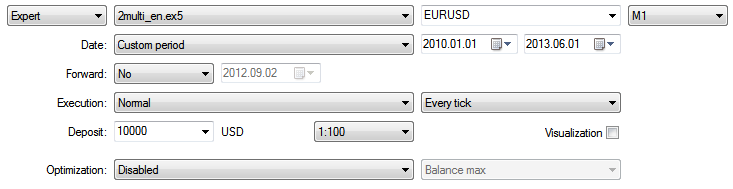Fig. 2. Strategy Tester settings

Results for strategy A, EURUSD: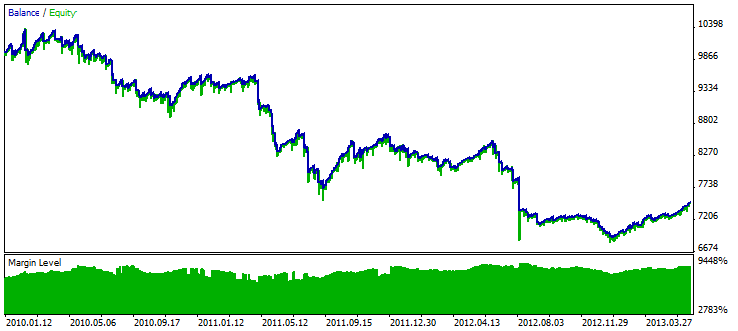Fig. 3. Test results for strategy A, EURUSD

Results for strategy A, GBPUSD: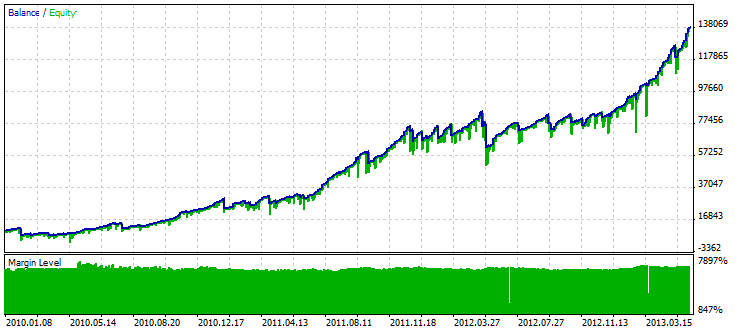Fig. 4. Test results for strategy A, GBPUSD

Results for strategy B, AUDUSD: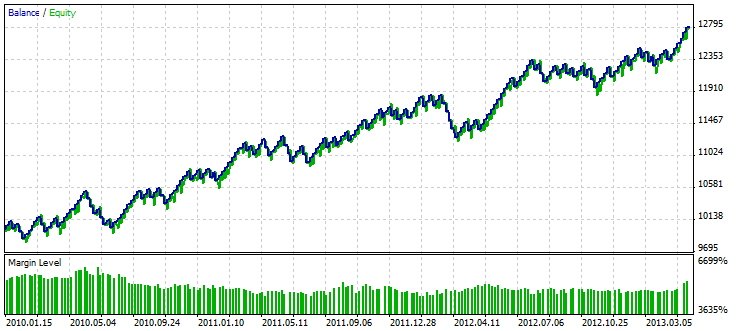Fig. 5. Test results for strategy В, AUDUSD

Results for strategy B, EURJPY: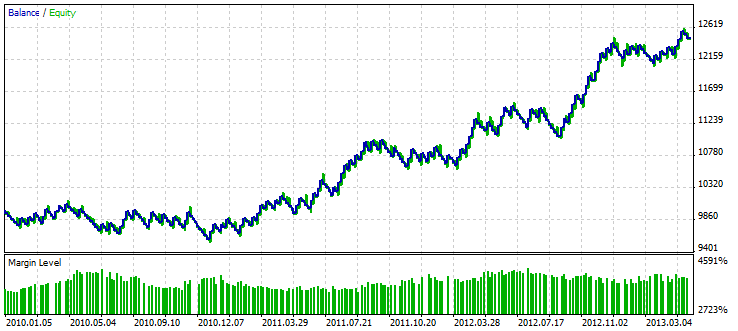Fig. 6. Test results for strategy В, EURJPY

Test results for all strategies and symbols: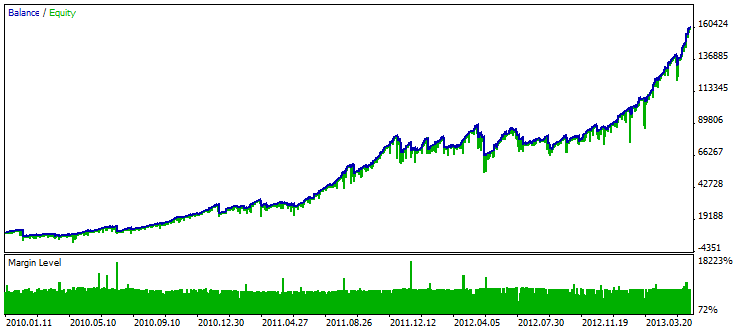Fig. 7. Test results for all strategies and symbols

### Conclusion

As a result, we have a convenient and simple structure of the multi-currency multi-system Expert Advisor in which you can place virtually any of your strategies.

Such an Expert Advisor allows you to better assess the efficiency of trading using all your strategies. It may also prove useful in case only one Expert Advisor is allowed to work on a given account. The source code of the Expert Advisor is attached to the article to facilitate studying the above information.

Translated from Russian by MetaQuotes Ltd.
Original article: https://www.mql5.com/ru/articles/770

Attached files |
2multi_en.mq5 (30.27 KB)
Last comments | Go to discussion (11)| 2 Sep 2014 at 03:15

Hello.

This code of EA is exactly what I'm looking for.

However, I'm doing it semi-auto with my custom EA with 15 symbols

though, I'm only controlling 2-3 at most. Would there be any version for

MT4?

Thanks.| 2 Sep 2014 at 03:18

Furthermore, I'm not using any indicators; my account equity is my indicator...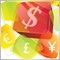| 13 Dec 2014 at 08:13
I tested strategy A for GBPUSD for same period with same settings in mt4 but my results are not same. Any idea why?| 19 Aug 2018 at 06:10

i have a simple question here.  If, say, the code within the OnTimer takes more than 1-second to execute on average (such as 2 or 3 seconds), should i change the EventSetTimer within OnInit to a higher value (such as 5 or 6 seconds) ?  Thanks.

==========================================

int OnInit()

{

//--- Set event generation frequency

EventSetTimer(1); // 1 second

// ...

return(0);

}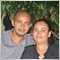| 23 Sep 2020 at 07:18
How to consult the floating profit? The open volume? Number of Positions?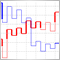Indicator for Kagi Charting
The article proposes Kagi chart indicator with various charting options and additional functions. Also, indicator charting principle and its MQL5 implementation features are considered. The most popular cases of its implementation in trading are displayed - Yin/Yang exchange strategy, pushing away from the trend line and consistently increasing "shoulders"/decreasing "waists".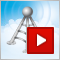Video tutorial: MetaTrader Signals Service
In just 15 minutes, this video tutorial explains what MetaTrader Signals Service is, and demonstrates in great detail how to subscribe to trade signals and how to become a signal provider in our service. By watching this tutorial, you will be able to subscribe to any trading signal, or publish and promote your own signals in our service.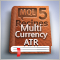MQL5 Cookbook: Developing a Multi-Symbol Volatility Indicator in MQL5
In this article, we will consider the development of a multi-symbol volatility indicator. The development of multi-symbol indicators may present some difficulties for novice MQL5 developers which this article helps to clarify. The major issues arising in the course of development of a multi-symbol indicator have to do with the synchronization of other symbols' data with respect to the current symbol, the lack of some indicator data and the identification of the beginning of 'true' bars of a given time frame. All of these issues will be closely considered in the article.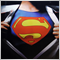Raise Your Linear Trading Systems to the Power
Today's article shows intermediate MQL5 programmers how they can get more profit from their linear trading systems (Fixed Lot) by easily implementing the so-called technique of exponentiation. This is because the resulting equity curve growth is then geometric, or exponential, taking the form of a parabola. Specifically, we will implement a practical MQL5 variant of the Fixed Fractional position sizing developed by Ralph Vince.